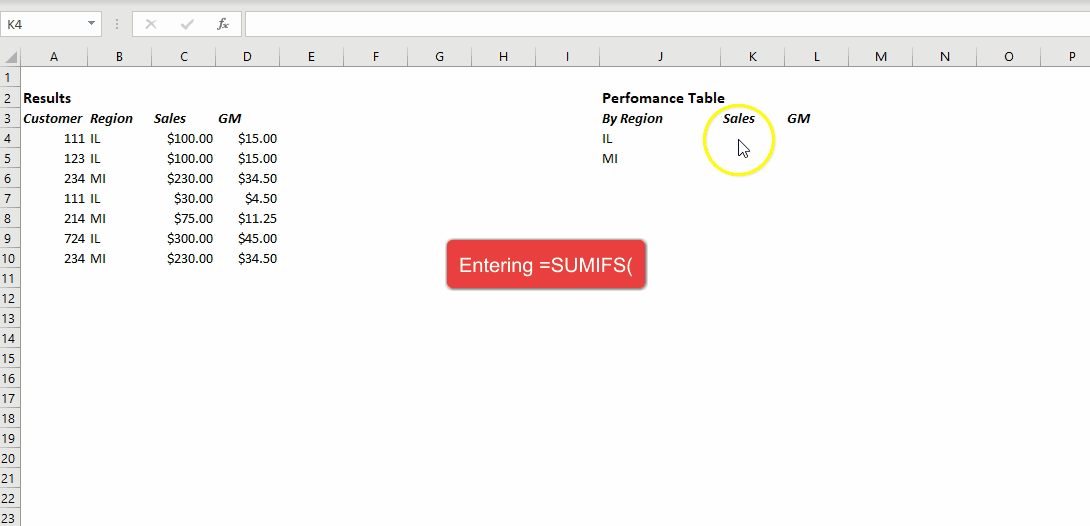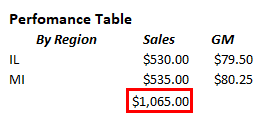top of page
Search

# SUMIFS & SUMSUMIFS adds value in an array provided all arguments are met.

SUMIFS has 3 arguments:

sum_range:

A table range of values which will be added together.

criteria_range:

A table range where values "targets" reside.

criteria:

Is a value that must be met for addition to happen.

In the example above, a function works by taking column number from sum_range input (column C) and assigning row number by matching value of IL (J4) to value specified in criteria_range (column B). The matches for value in J4 in column B are rows 4,5,7,9. These row values are combined with a column value from sum_range, which is column C. The final value of cells to be added together are, C4,C5,C7,C9.

References are locked by pressing F4 or entering \$ sign beside the column or range indicators. This is done to quickly copy the formula as it is dragged. During dragging down, a formula will not change.

A simpler version of the same function is a SUM function.

SUM functions adds together specified values.

The function has only one argument:

number1:

Lists values or range of values which will be added together.In the example above, we added two regions together. This could be achieved two different ways:

Directly type in values: =SUM(530,535)

Directly reference cells =SUM(K4,K5)

List a range =SUM(K4:K5)

All three examples would give the same result.

33 views

See All
bottom of page